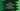# Java program to check if a number is Pronic or Heteromecic### Java program to check if a number is Pronic/Heteromecic number or not :

A number is called a Pronic number or Heteromecic number if it is equal to the product of two consecutive numbers. For example, 9 * 10 = 90, so 90 is a Pronic number.Our program will take the input from the user and check if it is Pronic.Let’s take a look at the program first :

### Java Program :

``````import java.util.Scanner;

public class Main {

public static void main(String[] args) {
//1
Scanner sc = new Scanner(System.in);
int no;
boolean flag = false;

//2
System.out.println("Enter a number to check if it is a Pronic or not : ");
no = sc.nextInt();

//3
for (int i = 0; i <= no; i++) {

//4
if (i * (i + 1) > no) {
break;
}

//5
if (i * (i + 1) == no) {
System.out.println("Number " + no + " is a Pronic number.");
flag = true;
break;
}
}

//6
if (!flag) {
System.out.println("Number " + no + " is not a Pronic number.");
}

}

}``````

### Explanation :

1. Create one Scanner object to read user-input values. One integer variable no to save the input number and one boolean flag to indicate if the number is detected as Pronic,i.e. it will be true if detected.
2. Ask the user to enter a number. Read it and save it in variable no.
3. Run one for loop from 0 to the no.
4. Check if the product of the current value and (current value + 1) is greater than the no or not.If yes, means all other numbers more than this number will not be equal to the user-input number. So, break from the loop.
5. If the product of the current value and (current value + 1) is equal to the user-input no, means this is a Pronic number. So, print out the value and mark the value of flag as true. Move out of the loop using break.
6. Check if the value of flag is ‘false’ or not.If ‘false’,print out that this number is not a Pronic number.

### Sample Output :

``````Enter a number to check if it is a Pronic or not :
0
Number 0 is not a Pronic number.

Enter a number to check if it is a Pronic or not :
112
Number 112 is not a Pronic number.

Enter a number to check if it is a Pronic or not :
306
Number 306 is a Pronic number.``````